# Drawing Curves

 [ LiB ]

Curves can get tricky because the technique it takes to draw them is one of the most difficult parts of using internal drawing commands. In ActionScript, you can specify a curve with endpoints and a control point. You would assign the two endpoints just as you would with a regular line. The control point is then assigned depending where you want the line to curve.

See Figure 7.8 before reading on; it's a figure showing the relationship between a curve and its control point. The control point is allowed to be anywherebut remember, wherever you chose to place it, that's the direction where the line will curve to.

##### Figure 7.8. Showing off the relationship between the endpoints and the control point of a curve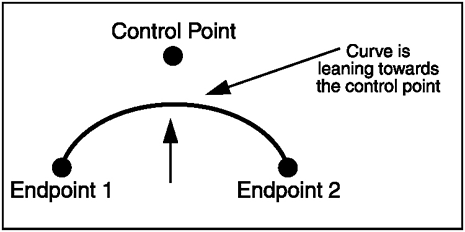Figure 7.9 shows a screen shot of the project file GDA_PROG7.7.fla. It demonstrates a curve that is drawn from (0, 100) to (320, 100). So why does the line curve up? There was a control point assigned at (160, 0)that's why it bends towards the middle-top.

##### Figure 7.9. Showing off a curve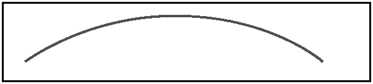So what would happen if the line's control point was (0, 0) or (320, 200)? Go ahead and see for yourself in Figure 7.10 and Figure 7.11.

##### Figure 7.10. Control point set at (0, 0)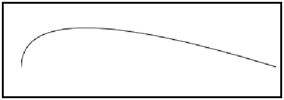##### Figure 7.11. Control point set at (320, 200)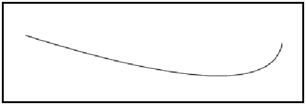Now you know we can draw curves with the minimum of three pointsthe two endpoints and the control point. What command can we use for this fabulous curve? Why, the curveTo command, of course. Here's its prototype:

` myMovieClip.curveTo (controlX, controlY, anchorX, anchorY) `

Just like the other line commands, curveTo draws from the previous point used (or the point that was given to moveTo ).

The controlx parameter specifies the x position relative to the registration point of the parent Movie Clip. In English, that would mean that controlx is the x value of where the control point will be. And of course, controly is the y value that completes the pair. These coordinates are the point that dictates how the curve actually curves.

anchorx and anchory are the endpoints of the curve. This coordinate is a point where the curve will end and where you can continue creating other curves.

NOTE

NOTE

If you are baffled by where you should place your points, take heed: there is nothing wrong with a little trial and error. Experimentation is part of perfection .

I think you are ready for another listing. The following listing creates the representation of a trigonometric function, sine. Check out the project file GDA_PROG7.8.fla.

` // Game Development with ActionScript // By Lewis Moronta (c) 2003 // Demo using ActionScript Curves. // Set the line style lineStyle( 0, 0x0000FF);  // Set the first point (internally) moveTo( 0, 100 ); // Setup the control point // and endpoint of the line. curveTo( 80, 0, 160, 100 ); curveTo(240, 200, 320, 100); `

The listing's output appears in Figure 7.12.

##### Figure 7.12. Simulated sine wave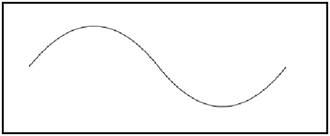By looking at the code, you can quickly see how simple this program really is. The first two lines are the common things we've been doing in this chapter so far:

` // Set the line style lineStyle( 0, 0x0000FF); // Set the first point (internally) moveTo( 0, 100 ); `

As you can see, I set the color to blue and I have hairline thickness on the curves. The moveTo command set the next point to (0, 100).

There are two curve methods here; check out the first one:

` // Setup the control point // and endpoint of the line. curveTo( 80, 0, 160, 100 ); `

Before we move on, you should know that I moved Flash's "virtual pen" to (0, 100) using the moveTo command.

As the first pair of parameters are the control point's location, you can already tell that this curve is going to bend up. I chose this point so that the line would curve from the center up.

As for the endpoints, I chose points that are centered on the stage. This caused a nice curve up and then down to the middle, where I drew another curvelet's talk about that one now. Take a look:

` curveTo(240, 200, 320, 100); `

As the last point was the center of the stage, (160, 100), the line is drawn from this point while curving to (240, 200), its endpoint being (320, 100). As the control point is beneath both of its endpoints, you already know that the curve bends down then comes back up.

And that's all it takes to make complicated curve shapes .

 [ LiB ]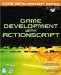Game Development with ActionScript
ISBN: 1592001106
EAN: 2147483647
Year: 2004
Pages: 162
Authors: Lewis Moronta